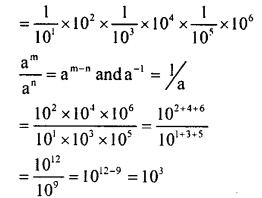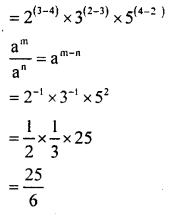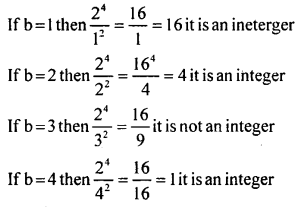# KSEEB Solutions for Class 8 Maths Chapter 10 Exponents Ex 10.3

In this chapter, we provide KSEEB SSLC Class 8 Maths Chapter 10 Exponents Ex 10.3 for English medium students, Which will very helpful for every student in their exams. Students can download the latest KSEEB SSLC Class 8 Maths Chapter 10 Exponents Ex 10.3 pdf, free KSEEB SSLC Class 8 Maths Chapter 10 Exponents Ex 10.3 pdf download. Now you will get step by step solution to each question.

### Karnataka State Syllabus Class 8 Maths Chapter 10 Exponents Ex 10.3

Question 1.
Simplify:
(i) 10-1 x 102 x 10-3 x 104 x 105 x 106
(ii) 23×32×5433×52×24
(i) 10-1 x 102 x 10-3 x 104 x 105 x 106(ii) 23×32×5433×52×24Question 2.
Which is larger (34 × 23) or (25 × 32)
34 × 23 = 81 × 8 = 648
25 × 32 = 32 × 9 = 288
648 > 288
34 × 2> 25 × 32

Question 3.
Suppose ‘m’ and ‘n’ are distinct integers  3m×2n2m×3n  can be an integer? Give reasons.$\frac{3^{m} \times 2^{n}}{2^{m} \times 3^{n}} = \frac{3^{m-n}}{2^{m-n}}$
If ‘m’ and ‘n’ are two distinct integers.
Then 3(m-n) is always on an odd number and 2(m-n) is always an even number.
If an odd number is divided, by an even number the quotient is not an integer.
Therefore it is’ not an integer.

Question 4.
Suppose b is a positive integer such that is also an integer. What are the possible values of b.If b > 4 the denominator e×ceeds the numerator and we get a fraction.
∴ The possible values of b are 1, 2, and 4.

All Chapter KSEEB Solutions For Class 8 maths

—————————————————————————–

All Subject KSEEB Solutions For Class 8

*************************************************

I think you got complete solutions for this chapter. If You have any queries regarding this chapter, please comment on the below section our subject teacher will answer you. We tried our best to give complete solutions so you got good marks in your exam.

If these solutions have helped you, you can also share kseebsolutionsfor.com to your friends.

Best of Luck!!